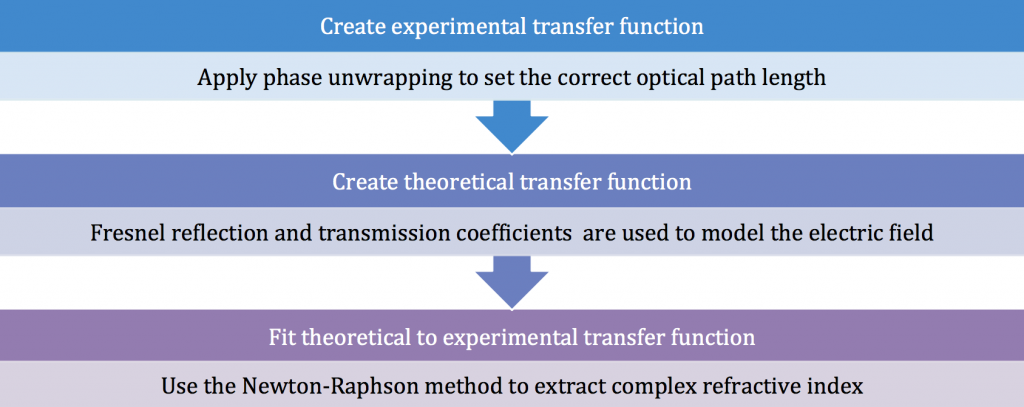# Parameter Extraction Algorithm

## Transfer Function based refractive index extraction algorithm for THz-TDS

The algorithm uses a transfer function to extract a refractive index from a slab material. It works for THz time domain spectroscopy measurements that have been taken in a transmission geometry. For inputs the algorithm requires; a time domain scan of a sample, a time domain reference scan, and the thickness of the sample. The algorithm will output the complex refractive index of the sample.

• The complex refractive index of a material is determined by fitting a theoretically calculated transfer function to the experimental data.
• The calculation of the theoretical transfer function is done using Fresnel coefficients
• The calculation of the experimental transfer function is the division in frequency of the signal obtained with the same and a reference scan without the sample
• Complex refractive index can be used to determine material properties such as relative permittivity, conductivityOur algorithms are free, now we are developing also machine learning algorithms to improve on the accuracy of normal transfer function based algorithms.